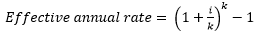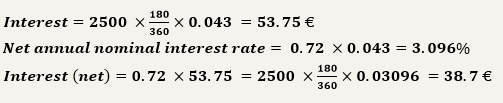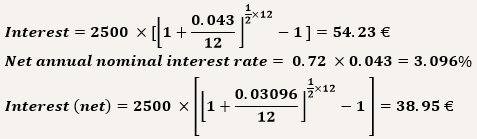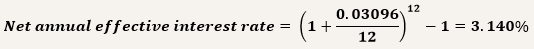# Interest rates

The remuneration of a time deposit – interest – depends on the interest rate that the credit institution practices in that deposit, the amount applied, the term of deposit and the tax rate that is levied on the interest paid by the institution.

The comparison of remunerations offered in applications in different currencies only makes sense if these remunerations are expressed in a common currency.

## Gross and net interest rate

The interest rate of deposit is called the gross annual nominal interest rate, which is:

• An annual rate – it expresses the remuneration of the deposit for the period of one year. To calculate interest on a deposit with a term other than one year, it is necessary to calculate the rate proportional to that period;

• A nominal rate – it does not vary according to inflation;

• A gross rate – it does not consider the tax that is levied on interest;

• Simple interest rate – it does not consider the capitalisation of interest that can be paid over the period.

### Tax applicable to interest on deposits

Interest paid by credit institutions on current or time deposits is subject to the payment of taxes.

If the depositor is a natural person, the personal income tax rate (IRS) applies:

• 28% for natural persons with tax residence in mainland Portugal and Madeira;

• 22.4% for natural persons with tax residence in the Azores.

In the case of companies, the corporate income tax rate (IRC) applies:

• 25% for companies with tax residence in mainland Portugal and Madeira;

• 20% for companies with tax residence in the Azores.

The net annual nominal interest rate incorporates the withholding tax (28% if there is no special regime) and corresponds to the interest that the customer receives.

The net annual nominal interest rate is greater than the gross annual nominal interest rate: Net annual nominal interest rate = 0.72 x Gross annual nominal interest rate.

### Example

A deposit of 2500 euros, applied for one year at a gross annual nominal interest rate of 4.3%, generates an interest at the end of the period of:

Interest = 2500 × 0.043 = 107,5 €

If the deposit of 2500 euros is applied for a different period of the year, for example for 180 days at a gross annual nominal interest rate of 4.3%, it generates an interest at the end of the period of:

Interest = 2500 × (180/360) × 0.043 = 53,75 €

If a withholding tax rate of 28% is applied, at the end of the period the initial capital and 72% of the interest generated will be credited to the current account.

Note: The interest accruing shall be calculated using Actual/360 method of calculation, the general money market convention in euros.

### Nominal and real interest rate

In order for the amount applied in deposits not to lose value over time, the interest received must compensate for the developments in the general price level, i.e. inflation.

The inflation-adjusted nominal interest rate is the real interest rate and corresponds to the difference between the net annual nominal interest rate and the inflation rate.

If the real interest rate is positive, the interest received on the deposit outweighs the developments in prices and the savings are gaining value because it is possible to buy a greater quantity of goods and services.

### Simple and compound interest rate

The nominal interest rate is a simple interest rate, since it considers that the amount applied in the time deposit remains constant over time and that the interest is paid in the associated current account.

Some time deposits allow for the capitalisation of interest, either automatically or at the customer’s initiative. This means that the interest earned in each period is added to the initial capital, constituting a new capital (greater than the initial capital), which will also be remunerated (compound interest).

While simple interest grows proportionately over time, compound interest grows more than proportionately over time.

### Example

A 2-year deposit of 2500 euros, with annual payment of interest at a gross annual nominal interest rate of 3%:

(1) At the end of the first year,

Interest = 2500 × 0.03 =75 €

Interest (net) = 0.72 × 75 = 54 €

This first payment is equal, whether interest is simple or compound.

(2) At the end of the second year,

Simple interest:

Interest = 2500 × 0.03 =75 €

Interest (net) = 0.72 ×75= 54 €

Compound interest:

Capital = 2500 + 54 = 2554 €

Interest = 2554 × 0.03 = 76.62 €

Interest (net) = 0.72 × 76.62 = 55.17 €

(3) In the two years,

Simple interest: Interest received = 54 + 54 = 108 €

Compound interest: Interest received = 54 + 55.17 = 109.17 €

In all, an additional 1.17 euros are received in the case of compound interest.

In time deposits with simple interest, the comparison between the remuneration of different time deposits should be made based on the gross annual nominal interest rate. In time deposits with compound interest, the comparison must take into account the effective annual rate, which includes the effect of capitalising interest.

The effective interest rate is all the greater the greater the number of interest capitalisations:, where i= annual interest rate and k = number of times the deposit pays interest during the year.

The net annual effective interest rate is equal to the effective annual rate minus the tax charged on interest.

### Example

A 180-day deposit of 2500 euros, at a gross annual nominal interest rate of 4.3%:

(1) If interest is simple,(2) If interest is compound and interest is received monthly (k = 12),This interest amount is different from € 54.23 less tax (€ 54.23 x € 0.72 = € 39.05), since the retention is made monthly, whenever interest is calculated.

The net annual effective interest rate associated with this application is:In the case of deposits for periods of less than one year, it should be taken into account that the calculation of the net annual effective interest rate presupposes reinvestment (of capital and interest) at the same rate during a period of one year, which is not always possible (e.g. if the deposit cannot be renewed on expiry, under the same conditions).

In the case of automatically renewable deposits at the end of the deposit period, it is important that the customer be aware of the interest rate that will be applied by the credit institution at the time of renewal.